# Statistical Analysis

## 1. Introduction

MetaboAnalystR, in parallel with the webserver, provides a comprehensive suite of statistical analyses to perform on a user-uploaded data set. While researchers may have several goals for their metabolomics data, the ultimate goal is to identify any significant metabolite/s indicitive of a disease state, drugs, diet, environment, geographical location, etc.

The standard workflow for statistical analysis is as follows:

Processed metabolomic data -> Univariate analysis -> Multivariate analysis -> Biological interpretation

Univariate tests differ from multivariate tests in that they assess the importance of each variable seperately. Meanwhile, multivariate tests assess two or more variables at once, while also taking into consideration the relationship between the variables. Finally, biological interpretation provides a biological context for the significant metabolites identified using univariate/multivariate methods. Below, we will discuss the various statistical methods in greater detail. For the tutorial, we will be using a dataset consisting of concentrations of 77 urine samples from cancer patients (cachexic vs. control) measured by 1H NMR - Eisner et al. 2010.

## 2. Univariate Methods

To begin the SA module, we will start with identifying important features from the data set using various univariate tests, including classical methods such as the Student’s t-test and ANOVA, as well as other methods such as the volcano plot and correlation analysis.

``````# Load MetaboAnalystR
library(MetaboAnalystR)

# Clean global environment
rm(list = ls())``````
``````library(MetaboAnalystR)
mSet<-InitDataObjects("conc", "stat", FALSE);``````
``````## Starting Rserve:
##  /opt/R/4.2.2/lib/R/bin/R CMD /opt/R/4.2.2/lib/R/library/Rserve/libs//Rserve --no-save
##
##  "MetaboAnalyst R objects initialized ..."``````
``````mSet<-Read.TextData(mSet, "https://www.xialab.ca/api/download/metaboanalyst/human_cachexia.csv", "rowu", "disc");
mSet<-SanityCheckData(mSet);``````
``````##   "Successfully passed sanity check!"
##   "Samples are not paired."
##   "2 groups were detected in samples."
##   "Only English letters, numbers, underscore, hyphen and forward slash (/) are allowed."
##   "<font color=\"orange\">Other special characters or punctuations (if any) will be stripped off.</font>"
##   "All data values are numeric."
##   "A total of 0 (0%) missing values were detected."
##   "<u>By default, missing values will be replaced by 1/5 of min positive values of their corresponding variables</u>"
##   "Click the <b>Proceed</b> button if you accept the default practice;"
##  "Or click the <b>Missing Values</b> button to use other methods."``````
``````mSet<-ReplaceMin(mSet);
mSet<-PreparePrenormData(mSet);
mSet<-Normalization(mSet, "NULL", "LogNorm", "MeanCenter", "S10T0", ratio=FALSE, ratioNum=20);``````
``##  77 63``
``````mSet<-PlotNormSummary(mSet, "norm_0_", format ="png", dpi=72, width=NA);
mSet<-PlotSampleNormSummary(mSet, "snorm_0_", format = "png", dpi=72, width=NA);``````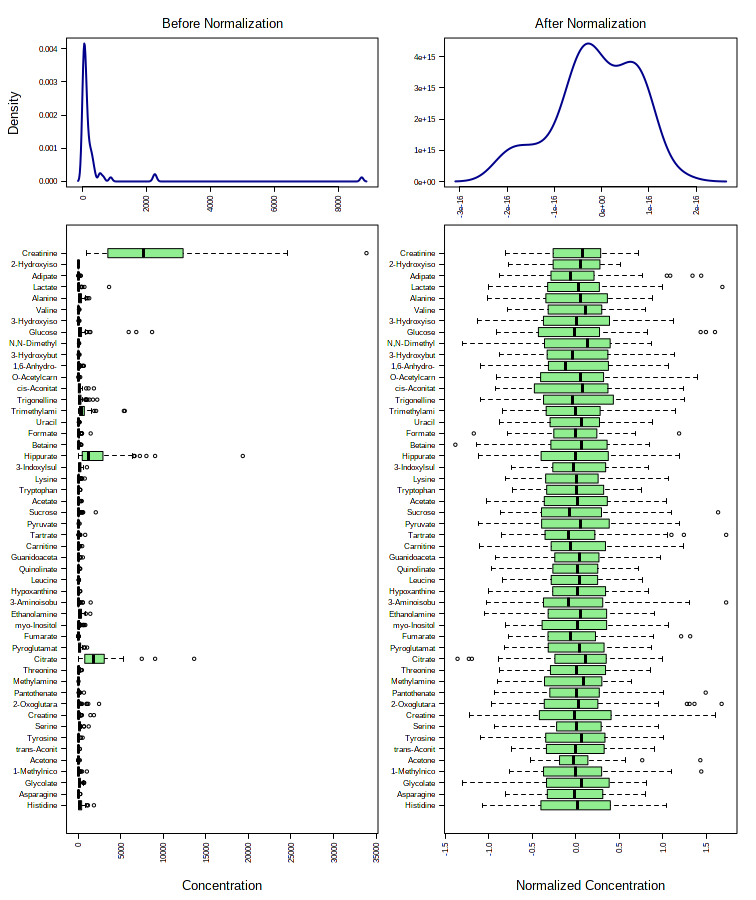Figure 1. Normalization results.

### 2.1 Fold-change analysis

The goal of fold-change (FC) analysis is to compare the absolute value of change between two group means. Since column-wise normalization (i.e. log transformation, mean-centering) will significantly alter absolute values, FC is calculated as the ratio between two group means using the data before column-wise normalization was applied.

For paired analysis, the program first counts the number of pairs with consistent change above the given FC threshold. If this number exceeds a given count threshold, the variable will be reported as significant.

``````# Perform fold-change analysis on uploaded data, unpaired
mSet<-FC.Anal(mSet, 2.0, 0, FALSE)

# Plot fold-change analysis
mSet<-PlotFC(mSet, "fc_0_", "png", 72, width=NA)

# To view fold-change
mSet\$analSet\$fc\$fc.log``````
``````## 1,6-Anhydro-beta-D-glucose       1-Methylnicotinamide
##                   0.888690                  -0.052019
##            2-Aminobutyrate       2-Hydroxyisobutyrate
##                   1.312700                   0.633520
##             2-Oxoglutarate         3-Aminoisobutyrate
##                   1.098400                   1.329100
##          3-Hydroxybutyrate       3-Hydroxyisovalerate
##                   1.563700                   1.164900
##           3-Indoxylsulfate     4-Hydroxyphenylacetate
##                   0.857170                   0.263810
##                    Acetate                    Acetone
##                   1.266100                   0.664560
##                   1.952900                   1.141300
##                 Asparagine                    Betaine
##                   0.852650                   1.004000
##                  Carnitine                    Citrate
##                   0.994090                   0.883620
##                   Creatine                 Creatinine
##                   1.763900                   0.932160
##              Dimethylamine               Ethanolamine
##                   1.120000                   0.729170
##                    Formate                     Fucose
##                   1.150600                   0.918770
##                   Fumarate                    Glucose
##                   1.262700                   2.553000
##                  Glutamine                    Glycine
##                   1.166100                   0.869890
##                  Glycolate             Guanidoacetate
##                   0.657790                   0.506100
##                  Hippurate                  Histidine
##                   1.075800                   1.013100
##               Hypoxanthine                 Isoleucine
##                   0.375470                   0.420550
##                    Lactate                    Leucine
##                   1.726900                   1.205400
##                     Lysine                Methylamine
##                   0.442780                   0.901220
##            Methylguanidine        N,N-Dimethylglycine
##                   0.517770                   1.342900
##          O-Acetylcarnitine               Pantothenate
##                   1.270300                  -0.397700
##              Pyroglutamate                   Pyruvate
##                   1.180400                   1.096200
##                Quinolinate                     Serine
##                   1.090600                   1.007700
##                  Succinate                    Sucrose
##                   1.416200                   1.432600
##                   Tartrate                    Taurine
##                   0.720000                   1.032700
##                  Threonine               Trigonelline
##                   0.990220                   1.460400
##     Trimethylamine N-oxide                 Tryptophan
##                   1.077700                   0.967880
##                   Tyrosine                     Uracil
##                   0.953700                   0.207270
##                     Valine                     Xylose
##                   1.178900                   1.194000
##              cis-Aconitate               myo-Inositol
##                   1.589400                   1.537500
##            trans-Aconitate         pi-Methylhistidine
##                   0.811730                   0.771640
##        tau-Methylhistidine
##                   0.708800``````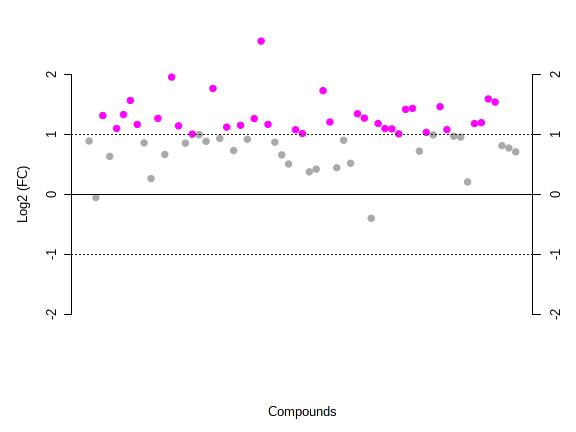Figure 2. Fold change analysis results.

### 2.2 T-Test

MetaboAnalystR supports various options for performing T-test analysis. Users can select the analysis type (paired), the group variance (equal.var), whether the test is parametric or non-parametric (nonpar), and the adjusted p-value (FDR) cut-off (threshp).

Note, for a large data set (> 1000 variables), both the paired information and the group variance will be ignored, and the default parameters will be used for t-tests to save computational time. If you choose non-parametric tests (Wilcoxon rank-sum test), the group variance will be ignored.

``````# Perform T-test (parametric)
mSet<-Ttests.Anal(mSet, nonpar=F, threshp=0.05, paired=FALSE, equal.var=TRUE, "fdr", FALSE)``````
``## Loading required package: memoise``
``````##  "Performing regular t-tests ...."
##  "A total of 53 significant features were found."``````
``````# Plot of the T-test results
mSet<-PlotTT(mSet, imgName = "tt_0_", format = "png", dpi = 72, width=NA)``````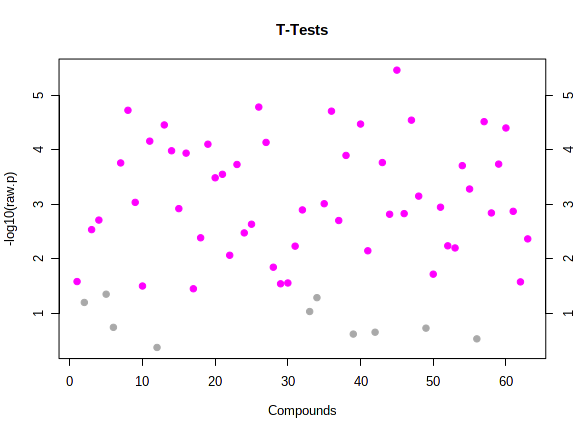Figure 3. T test analysis results.

The t test results for MS1 feature table can be used for function analysis.

## 2.3 Volcano Plot

The volcano plot is a combination of fold change and t-test values. Note, for unpaired samples, the x-axis is log2(FC). For paired analysis, the x-axis is number of significant counts. The y-axis is -log10(p.value) for both cases, and can be based on raw or FDR adjusted p values from the t-tests. In Volcano.Anal, users can specify if the data are paired, the FC threshold, the comparison type, the signigicant count threshold if data are paired, whether the test is parametric or non-parametric, the p-value threshold, and the group variance.

``````# Perform the volcano analysis
mSet<-Volcano.Anal(mSet, FALSE, 2.0, 0, F, 0.1, TRUE, "raw")``````
``##  "A total of 57 significant features were found."``
``````# Create the volcano plot
mSet<-PlotVolcano(mSet, "volcano_0_", 1, 0, format ="png", dpi=72, width=NA)``````
``## Loading required package: ggplot2``
``## Warning: Removed 30 rows containing missing values (`geom_text_repel()`).``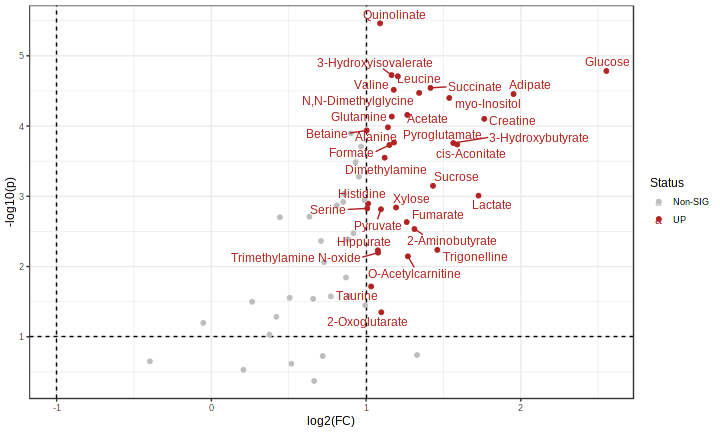Figure 4. Results of volcano analysis.

## 2.4 One-way Analysis of Variance (ANOVA) - ONLY FOR MULTI-GROUP ANALYSIS!

ANOVA can only be computed on data with more than one group. Please note that the below example uses data from a different example dataset, which contains more than 2 groups.

``````# Perform ANOVA
mSet <- ANOVA.Anal(mSet, F, 0.05, "fisher")

# Plot ANOVA
mSet <- PlotANOVA(mSet, "aov_0_", "png", 72, width=NA)``````

## 2.5 Correlation Analysis

To perform correlation analysis to evaluate the correlation between all features or samples, use PlotCorrHeatMap. Here, users must specify the mSet object, the dimensions to be correlated, the name of the heatmap that will be created, the output, dpi, and width of the image, the distance measure (Pearson, Spearman, or Kendall), to view the heatmap as an overview or detailed view, to fix the colour distribution, the colors, and whether or not to perform clustering. Please refer to the user manual for more details.

Note, the heatmap will only show correlations for a maximum of 1000 features. For larger datasets, only top 1000 features will be selected based on their interquantile range (IQR). When color distribution is fixed, you can potentially compare the correlation patterns among different data sets. In this case, you can choose “do not perform clustering” for all data set, or only to perform clustering on a single reference data set, then manually re-arranged other data sets according to the clustering pattern of the reference data set.

``````### OPTION 1 - Heatmap specifying pearson distance and an overview
mSet<-PlotCorrHeatMap(mSet, "corr_0_", "png", 72, width=NA, "col", "pearson", "bwm", "overview", F, F, 0.0)``````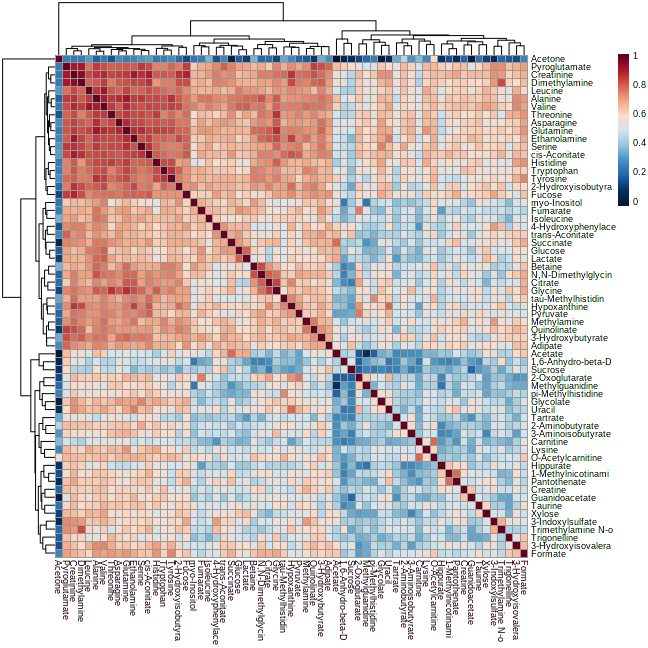Figure 5. Results of heatmap analysis.

The following command shows the detailed view (zoomed-in) of the above heatmap.

``````### OPTION 2 - Heatmap specifying pearson correlation and a detailed view
mSet<-PlotCorrHeatMap(mSet, "corr_1_", format = "png", dpi=72, width=NA, "col", "spearman", "bwm", "detail", F, F, 999)``````

## 2.6 Pattern Searching

Correlation analysis can be performed either against a given feature or against a given pattern. The pattern is specified as a series of numbers separated by “-”. Each number corresponds to the expected expression pattern in the corresponding group. For example, a 1-2-3-4 pattern is used to search for features that increase linearly with time in a time-series data with four time points (or four groups). The order of the groups is given as the first item in the predefined patterns. Indicate the mSet object, the distance measure (Pearson, Spearman, or Kendall), and the pattern to use.

``````# Perform correlation analysis on a pattern (a feature of interest in this case)
mSet<-FeatureCorrelation(mSet, "pearson", "1,6-Anhydro-beta-D-glucose")

# Plot the correlation analysis on a pattern
mSet<-PlotCorr(mSet, "ptn_3_", format="png", dpi=72, width=NA)``````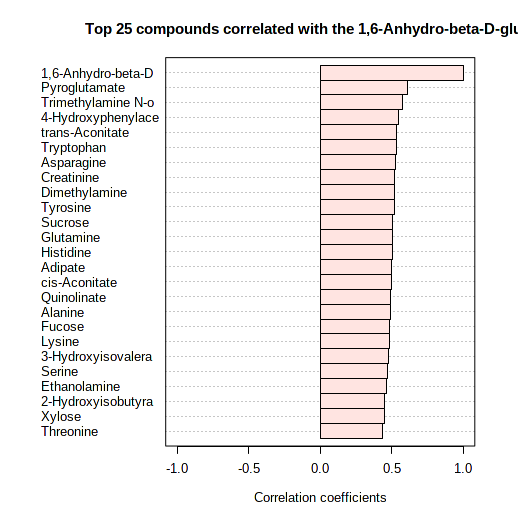Figure 6. Results of pattern search.

## 2.7 Principal Component Analysis (PCA)

To perform PCA, first use PCA.Anal. MetaboAnalystR has the options to create a scree plot (PlotPCAScree), score plot (PlotPCA2DScore or PlotPCA3DScore), loadings plot (PlotPCALoading), and biplot (PlotPCABiplot). For the score plots, users will create both a static as well as interactive 3D score plot. To view the interactive plot, type “mSet\$imgSet\$pca.3d” into your R console. Please refer to the user manual for details about each function.

``````# Perform PCA analysis
mSet<-PCA.Anal(mSet)

# Create PCA overview
mSet<-PlotPCAPairSummary(mSet, "pca_pair_0_", format = "png", dpi = 72, width=NA, 5)

# Create PCA scree plot
mSet<-PlotPCAScree(mSet, "pca_scree_0_", "png", dpi = 72, width=NA, 5)

# Create a 2D PCA score plot
mSet<-PlotPCA2DScore(mSet, "pca_score2d_0_", format = "png", dpi=72, width=NA, 1, 2, 0.95, 1, 0)

# Create a 3D PCA score plot
mSet<-PlotPCA3DScoreImg(mSet, "pca_score3d_0_", "png", 72, width=NA, 1,2,3, 40)

# Create a PCA Biplot
mSet<-PlotPCABiplot(mSet, "pca_biplot_0_", format = "png", dpi = 72, width=NA, 1, 2)``````
``````# View the 3D interactive PLS-DA score plot
mSet\$imgSet\$pca.3d``````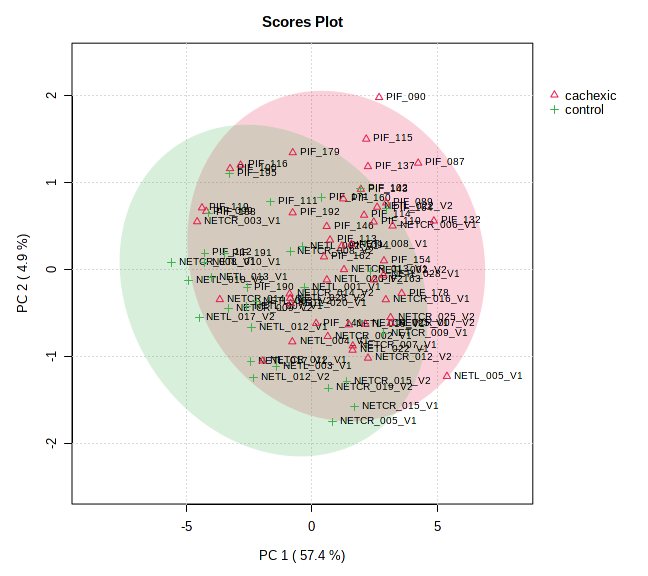Figure 7. PCA score plot.

## 2.8 Partial Least Squares - Discriminant Analysis (PLS-DA)

To perform PLS-DA, first use PLSR.Anal. MetaboAnalystR has the options to create a plot component pairs (PlotPLSPairSummary), score plot (PlotPLS2DScore), loadings plot (PlotPLSLoading), perform cross-validation and permutation (PLSDA.CV and PLSDA.Permut), and plot the results of the cross-validation and permutation (PlotPLS.Imp and PlotPLS.Permutation). For the score plots, users will create both a static as well as interactive 3D score plot. To view the interactive plot, type “mSet\$imgSet\$plsda.3d” into your R console. Please refer to the user manual for details about each function.

``````mSet<-PLSR.Anal(mSet, reg=TRUE)

mSet<-PlotPLSPairSummary(mSet, "pls_pair_0_", "png", 72, width=NA, 5)

mSet<-PlotPLS2DScore(mSet, "pls_score2d_0_", "png", 72, width=NA, 1,2,0.95,1,0)

mSet<-PlotPLS3DScoreImg(mSet, "pls_score3d_0_", "png", 72, width=NA, 1,2,3, 40)

mSet<-PLSDA.CV(mSet, "5", 5,5, "Q2")``````
``## Loading required package: lattice``
``````##
## Attaching package: 'caret'``````
``````## The following object is masked from 'package:MetaboAnalystR':
##
##     splsda``````
``## Loading required package: pls``
``````##
## Attaching package: 'pls'``````
``````## The following object is masked from 'package:caret':
##
##     R2``````
``````## The following object is masked from 'package:stats':
##
``````mSet<-PlotPLS.Classification(mSet, "pls_cv_0_", "png", 72, width=NA)

mSet<-PlotPLS.Imp(mSet, "pls_imp_0_", "png", 72, width=NA, "vip", "Comp. 1", 15, FALSE)

mSet<-PLSDA.Permut(mSet, 100, "accu")``````
``````##  "performing 100 permutations ..."
##  "Empirical p value: p = 0.02 (2/100)"``````
``mSet<-PlotPLS.Permutation(mSet, "pls_perm_1_", "png", 72, width=NA)``
``````# View the 3D interactive PLS-DA score plot
mSet\$imgSet\$plsda.3d``````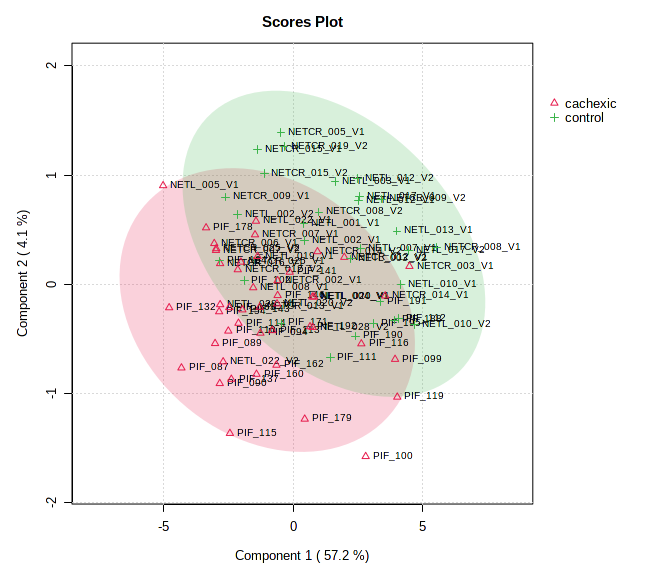Figure 8. PLS 2D score plot.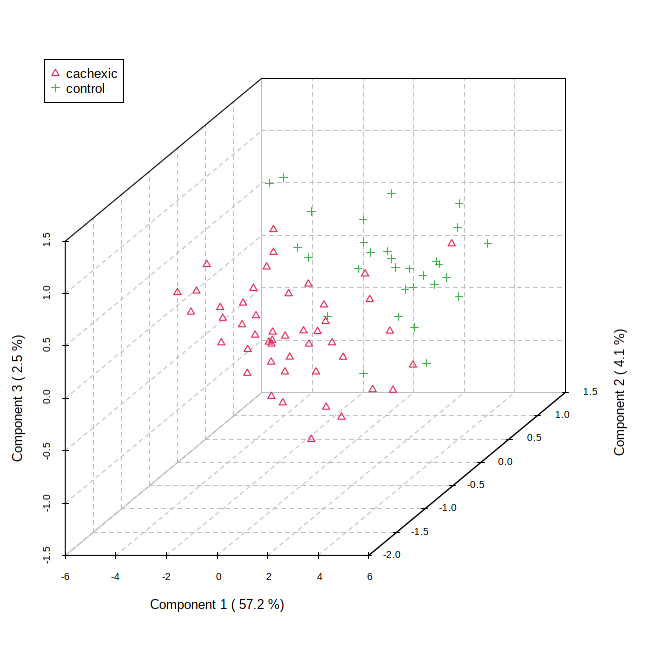Figure 9. PLS 3D score plot.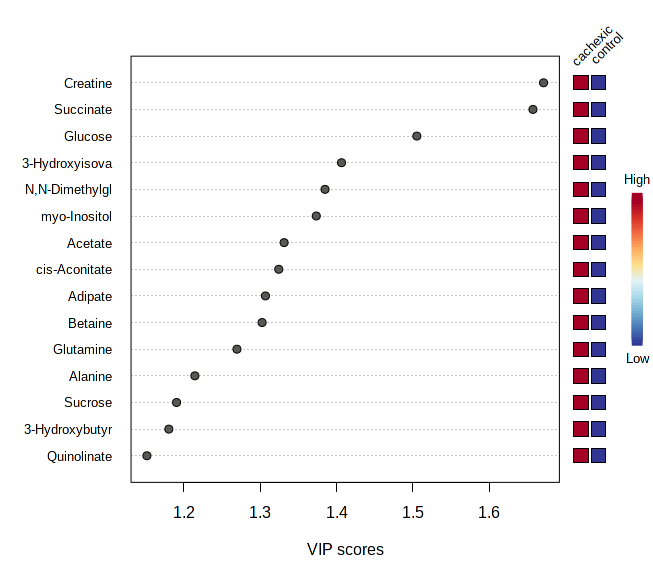Figure 10. PLS importance analysis results.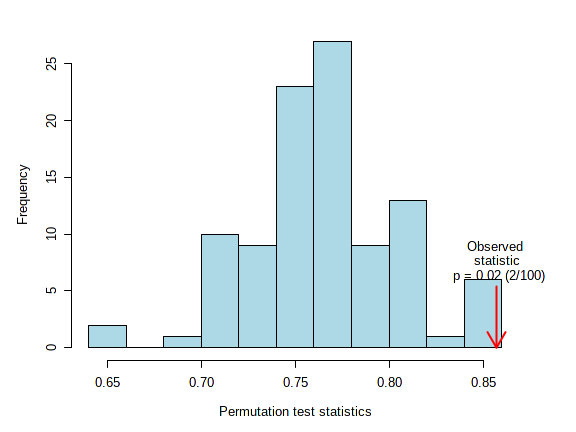Figure 11. PLS permutation test results.

## 2.9 Sparse Partial Least Squares - Discriminant Analysis (sPLS-DA)

The sparse PLS-DA (sPLS-DA) algorithm can effectively reduce the number of variables (metabolites) in high-dimensional metabolomics data to produce robust and easy-to-interpret models. Users can control the “sparseness” of the model by controlling the number of components included in the model and the number of variables within each component. More details can be found from Le Cao et. al 2011 (PMC3133555).

To evaluate the performance of the created sPLS-DA models for classification, use PlotSPLSDA.Classification. The performance of the sPLS-DA models are evaluated using cross validations (CV) with increasing numbers of components created using the specified number of the variables. Users can choose to use either 5-fold CVs or leave one out cross-validation (LOOCV). Please note that the results from 5-fold CV may change slightly due to random subsampling procedures.

``````# Perform sPLS-DA analysis
mSet<-SPLSR.Anal(mSet, 5, 10, "same", "Mfold", 5, T)

# Plot sPLS-DA overview
mSet<-PlotSPLSPairSummary(mSet, "spls_pair_0_", format = "png", dpi=72, width=NA, 5)

# Create 2D sPLS-DA Score Plot
mSet<-PlotSPLS2DScore(mSet, "spls_score2d_0_", format = "png", dpi=72, width=NA, 1, 2, 0.95, 1, 0)

# Create 3D sPLS-DA Score Plot
mSet<-PlotSPLS3DScoreImg(mSet, "spls_score3d_0_", format = "png", 72, width=NA, 1, 2, 3, 40)

``````# View the 3D interactive PLS-DA score plot